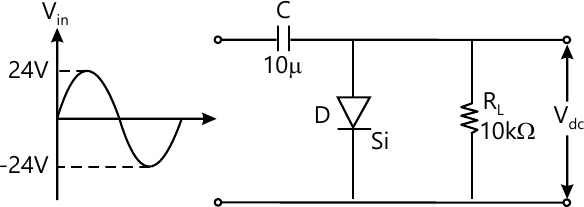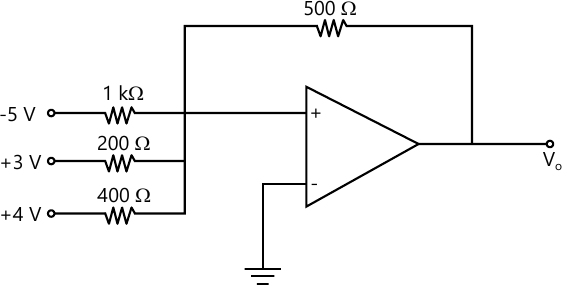MORE IN Basic Electronics Engineering (BXE)
SPPU First Year Engineering (Semester 2)
Basic Electronics Engineering (BXE)
December 2013
Total marks: --
Total time: --
INSTRUCTIONS
(1) Assume appropriate data and state your reasons
(2) Marks are given to the right of every question
(3) Draw neat diagrams wherever necessary

Answer any one question from Q1 & Q2
1 (a) Draw the circuit of series negative clipper and explain its operation along with the waveform.
6 M
1 (b) Explain working of transistor as a switch.
4 M
1 (c) Define current amplification factor for CC, CB, CE configuration.
2 M

2 (a) Draw the waveform across RL in clamping circuit.6 M
2 (b) Explain drain and transfer chara. of Enhancement type p-channel MOSFET.
6 M

Answer any one question from Q3 & Q4
3 (a) Draw and explain Internal block diagram of IC 555.
6 M
3 (b) Draw the schematic diagram & explain working of 4:1 mux and 1:4 demux.
6 M

4 (a) Find output voltage Vo of op-amp circuit shown in fig. Below:6 M
4 (b) State different types of counter & design 3bit negative edge triggered asynchronous down counter.
6 M

Answer any one question from Q5 & Q6
5 (a) Draw and explain operation of SCR using two transistor equivalent circuit.
6 M
5 (b) Draw and explain block diagram of instrumentation system.
7 M

6 (a) Draw constructional diagram and explain working of V-I characteristic of Diac.
6 M
6 (b) Draw & explain the construction and operation of LVDT.
7 M

Answer any one question from Q7 & Q8
7 (a) Explain the elements of communication system with the help of block diagram.
7 M
7 (b) What is baseband communication. Explain limitation of baseband communication & explain need for modulation.
6 M

8 (a) Write the expression of Amplitude modulation. Define modulation index & draw waveform of AM.
6 M
8 (b) Draw & explain block diagram of GSM system.
7 M

More question papers from Basic Electronics Engineering (BXE)